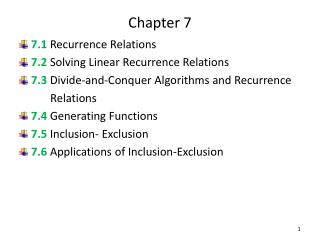Download PresentationChapter 7

Chapter 7 - PowerPoint PPT Presentation

Chapter 7. 7.1 Recurrence Relations 7.2 Solving Linear Recurrence Relations 7.3 Divide-and-Conquer Algorithms and Recurrence Relations 7.4 Generating Functions 7.5 Inclusion- Exclusion 7.6 Applications of Inclusion-Exclusion. 7.1 Recurrence Relations .I am the owner, or an agent authorized to act on behalf of the owner, of the copyrighted work described.
Download PresentationChapter 7

Download Policy: Content on the Website is provided to you AS IS for your information and personal use and may not be sold / licensed / shared on other websites without getting consent from its author.While downloading, if for some reason you are not able to download a presentation, the publisher may have deleted the file from their server.

- - - - - - - - - - - - - - - - - - - - - - - - - - E N D - - - - - - - - - - - - - - - - - - - - - - - - - -
Presentation Transcript
1. Chapter 7 • 7.1Recurrence Relations • 7.2 Solving Linear Recurrence Relations • 7.3Divide-and-Conquer Algorithms and Recurrence Relations • 7.4 Generating Functions • 7.5 Inclusion- Exclusion • 7.6Applications of Inclusion-Exclusion

2. 7.1 Recurrence Relations • Recurrence Relations • Modeling with Recurrence Relations

3. Recurrence Relations • The number of bacteria in a colony doubles every hour. If a colony begins with five bacteria , how many will be present in n hours? • Some of the counting problems that cannot be solved using the techniques discussed in Chapter 5 can be solved by finding relationships, called recurrence relations. • We will study variety of counting problems that can be modeled using recurrence relations. • We will develop methods in this section and in Section 7.2 for finding explicit formulae for the terms of sequences that satisfy certain types of recurrence relations.

4. Recurrence Relations • Example 1: Let {an} be a sequence that satisfies the recurrence relation an = an-1 – an-2for n=2, 3, 4, . . . And suppose that a0= 3and a1= 5. What are a2 and a3 ? • Example 2: Determine whether the sequence {an}, where an=3n for every nonnegative integer n, is a solution of the recurrence relation an=2an-1 –an-2 for n=2, 3, 4, . . ., Answer the same question where an= 2n and where an= 5?

5. Modeling with Recurrence Relations • Example 3: Compound Interest Suppose that person deposits \$10,000 in a savings account at a bank yielding 11% per year with interest compounded annually. • How much sill be in the account after 30 year? • Solution: Pn = Pn-1 +0.11Pn-1 = (1.11)Pn-1 • P1 = (1.11)P0 • P2 = (1.11) p1=(1.11)2 p0 • P3 = (1.11) P2=(1.11)3 P0 : • Pn = (1.11) Pn-1=(1.11)n P0 • Inserting n =30 into the formula Pn =(1.11)n 10,000 shows that after 30 year the account contains • P30 = (1.11)3010,000=\$228,922.97

6. Modeling with Recurrence Relations • Example 4: Rabbits and the Fibonacci Numbers consider this problem, which was originally posed by Leonardo Pisano, also Known as Fibonacci, in the thirteenth century in his book Liber abaci. A young pair of rabbits ( one of each sex) is placed on n island. • A pair of rabbits does not breed until they are 2 months old. After they are 2 months old, each pair of rabbits produces another pair each month, as shown in Figure 1. • Find a recurrence relation for the number of pairs of rabbits on the island after n months, assuming that no rabbits ever die.

7. Modeling with Recurrence Relations • Consequently, the sequence {fn} satisfies the recurrence relation fn =fn-1 + fn-2 ; f1=1, f2=1 FIGURE 1 Rabbits on an Island.

8. Modeling with Recurrence Relations • Example 5: The Tower of Hanoi A popular puzzle of the late nineteenth century invented by the French mathematician Édouard Lucas, called the Tower of Hanoi, consists of three pegs mounted on a board together with disks of different sizes. Initially there disks are placed on the first peg in order of size, with the largest on the bottom ( as shown in Figure 2). • The rules of the puzzle allow disks to be moved one at a time from one peg to another as long as a disk is never placed on the top of a smaller disk. • The goal of the puzzle is to have all the disks on the second peg in order of size, with the largest on the bottom. • Let {Hn} denote the number of moves needed to solve the Tower of Hanoi problem with n disks. Set up a recurrence relation for the sequence {Hn}

9. Modeling with Recurrence Relations FIGURE 2 The Initial Position in the Tower of Hanoi. FIGURE 3 An Intermediate Position in the Tower of Hanoi.

10. Modeling with Recurrence Relations • Example 6: Find a recurrence relation and give initial conditions for the number of bit strings of length n that do not have two consecutive 0s. How many such bit strings are there of length five? • Example 7: Codeword Enumeration A computer system considers a string of decimal digits a valid codeword if it contains an even number of 0 digits. For instance, 1230407869 is valid, whereas 120987045608 is not valid. Let an be the number of valid n-digit codewords. Find a recurrence relation for an.

11. Modeling with Recurrence Relations • Example 8: Find a recurrence relation for Cn, the number of ways to parenthesize the product of n+1 numbers, x0．x1．x2．. . .．xn, to specify the order of multiplication. For example ,C3=5 because there are five ways to parenthesize x0．x1．x2．x3 to determine the order of multiplication: • ((x0．x1) ．x2) ．x3 • (x0．(x1 ．x2))．x3 • (x0．x1) ．(x2 ．x3) • x0．((x1 ．x2) ．x3 ) • x0．(x1 ．(x2．x3))